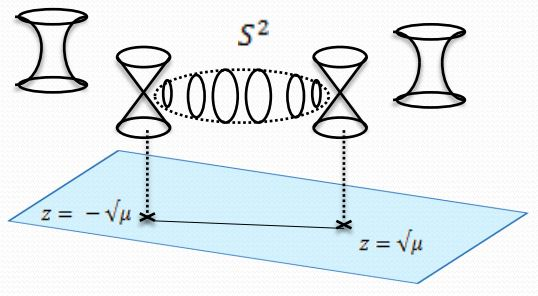# nLab duality between M/F-theory and heterotic string theory

Contents

This entry is about M-theory/F-theory compactified on K3-surfaces. For M-theory on MO9-planes see instead at Hořava-Witten theory.

### Context

#### Duality in string theory

duality in string theory

general mechanisms

string-fivebrane duality

string-string dualities

M-theory

F-theory

string-QFT duality

QFT-QFT duality:

# Contents

## Idea

F-theory$\,$KK-compactified” on an elliptically fibered K3 with a section is supposed to be equivalent to heterotic string theory KK-compactified on a 2-torus.

More generally, F-theory on a complex $n$-dimensional $X$ fibered $X\to B$ with elliptic K3-fibers is supposed to be equivalent to heterotic string theory on an elliptically fibered Calabi-Yau manifold $Z \to B$ of complex dimension $(n-1)$.

A detailed discussion of the equivalence of the respective moduli spaces is originally due to (Friedman-Morgan-Witten 97). A review of this is in (Donagi 98).

From the abstract of (Donagi 98).

The heterotic string compactified on an $(n-1)$-dimensional elliptically fibered Calabi-Yau $Z \to B$ is conjectured to be dual to F-theory compactified on an $n$-dimensional Calabi-Yau $X \to B$, fibered over the same base with elliptic K3 fibers. In particular, the moduli of the two theories should be isomorphic. The cases most relevant to the physics are $n=2$, $3$, $4$, i.e. the compactification is to dimensions $d=8$, $6$ or $4$ respectively. Mathematically, the richest picture seems to emerge for $n=3$, where the moduli space involves an analytically integrable system whose fibers admit rather different descriptions in the two theories.

## Aspects

### Singular locus of the elliptic fibration and 24 D7-branes

In passing from M-theory to type IIA string theory, the locus of any Kaluza-Klein monopole in 11d becomes the locus of D6-branes in 10d. The locus of the Kaluza-Klein monopole in turn (as discussed there) is the locus where the $S^1_A$-circle fibration degenerates. Hence in F-theory this is the locus where the fiber of the $S^1_A \times S^1_B$-elliptic fibration degenerates to the nodal curve. Since the T-dual of D6-branes are D7-branes, it follows that D7-branes in F-theory “are” the singular locus of the elliptic fibration.

$\array{ T &\longrightarrow& K3 \\ && \downarrow \\ && \mathbb{C}\mathbb{P}^1 }$

may be parameterized via the Weierstrass elliptic function as the solution locus of the equation

$y^2 = x^3 + f(z) x + g(z)$

for $x,y,z \in \mathbb{C}\mathbb{P}^1$, with $f$ a polynomial of degree 8 and $g$ of degree twelve. The j-invariant of the complex elliptic curve which this parameterizes for given $z$ is

$j(\tau(z)) = \frac{4 (24 f)^3}{27 g^2 + 4 f^3} \,.$

The poles $j\to \infty$ of the j-invariant correspond to the nodal curve, and hence it is at these poles that the D7-branes are located.

Since the order of the poles is 24 (the polynomial degree of the discriminant $\Delta = 27 g^2 + 4 f^3$, see at elliptically fibered K3-surfacesingular points) there are necessarily 24 D7-branes (Sen 96, page 5, Sen 97b, see also Morrison 04, sections 8 and 17, Denef 08, around (3.41), Douglas-Park-Schnell 14).

Under T-duality this translates to 24 D6-branes in type IIA string theory on K3 (Vafa 96, Footnote 2 on p. 6).

Notice that the net charge of these 24 D7-branes is supposed to vanish, due to S-duality effects (e.g. Denef 08, below (3.41)).

(This reminds one of the situation for the third stable homotopy group of spheres…)

For analogous discussion of 24 NS5-branes in heterotic string theory on K3 see Schwarz 97, around p. 50.

For more see at 24 branes transverse to K3.

### From M-branes to F-branes to heterotic strings and NS5-branes

from M-branes to F-branes: superstrings, D-branes and NS5-branes

M-theory on $S^1_A \times S^1_B$-elliptic fibrationKK-compactification on $S^1_A$type IIA string theoryT-dual KK-compactification on $S^1_B$type IIB string theorygeometrize the axio-dilatonF-theory on elliptically fibered-K3 fibrationduality between F-theory and heterotic string theoryheterotic string theory on elliptic fibration
M2-brane wrapping $S_A^1$double dimensional reduction $\mapsto$type IIA superstring$\mapsto$type IIB superstring$\mapsto$$\mapsto$heterotic superstring
M2-brane wrapping $S_B^1$$\mapsto$D2-brane$\mapsto$D1-brane$\mapsto$
M2-brane wrapping $p$ times around $S_A^1$ and $q$ times around $S_B^1$$\mapsto$$p$ strings and $q$ D2-branes$\mapsto$(p,q)-string$\mapsto$
M5-brane wrapping $S_A^1$double dimensional reduction $\mapsto$D4-brane$\mapsto$D5-brane$\mapsto$
M5-brane wrapping $S_B^1$$\mapsto$NS5-brane$\mapsto$NS5-brane$\mapsto$$\mapsto$NS5-brane
M5-brane wrapping $p$ times around $S_A^1$ and $q$ times around $S_B^1$$\mapsto$$p$ D4-brane and $q$ NS5-branes$\mapsto$(p,q)5-brane$\mapsto$
M5-brane wrapping $S_A^1 \times S_B^1$$\mapsto$$\mapsto$D3-brane$\mapsto$
KK-monopole/A-type ADE singularity (degeneration locus of $S^1_A$-circle fibration, Sen limit of $S^1_A \times S^1_B$ elliptic fibration)$\mapsto$D6-brane$\mapsto$D7-branes$\mapsto$A-type nodal curve cycle degeneration locus of elliptic fibration(Sen 97, section 2)SU-gauge enhancement
KK-monopole orientifold/D-type ADE singularity$\mapsto$D6-brane with O6-planes$\mapsto$D7-branes with O7-planes$\mapsto$D-type nodal curve cycle degeneration locus of elliptic fibration(Sen 97, section 3)SO-gauge enhancement
exceptional ADE-singularity$\mapsto$$\mapsto$$\mapsto$exceptional ADE-singularity of elliptic fibration$\mapsto$E6-, E7-, E8-gauge enhancement

(e.g. Johnson 97, Blumenhagen 10)

### Non-reducible heterotic $E_8$-gauge backgrounds

There are some F-theory backgrounds whose supposed dual in heterotic string theory involves an E8-principal connection which is not reducible to SemiSpin(16) (Distler-Sharpe 10, section 5), while in fact the traditional construction of the heterotic worldsheet theory only covers this case. In (Distler-Sharpe 10, section 7-8) it is argued that therefore a more general formulation of heterotic string theory needs to involve parameterized WZW models. See also at heterotic string – Properties – General gauge backgrounds and parameterized WZW models.

F-theory KK-compactified on elliptically fibered complex analytic fiber $\Sigma$

$dim_{\mathbb{C}}(\Sigma)$12345
F-theoryF-theory on CY2F-theory on CY3F-theory on CY4F-theory on CY5

### For type IIA and M-theory

The conjectured duality between type IIA string theory KK-compactified on K3 times an n-torus and heterotic string theory on the $(n+2)$-torus is originally due to

Review:

Further discussion:

Specifically in relation to the putative K-theory-classification of D-brane charge:

Specifically in M-theory on G2-manifolds:

Specifically in relation to Moonshine:

### For F-theory

Discussion for F-theory includes

Review of (Friedman-Morgan-Witten 97) is in

• Ron Donagi, ICMP lecture on heterotic/F-theory duality (arXiv:hep-th/9802093)

• Björn Andreas, $N=1$ Heterotic/F-theory duality, PhD thesis (pdf)

with more details in

The issue with non-reducible $E_8$-gauge connections is highligted in

On a subtlety in the application of the Narasimhan-Seshadri theorem in the duality:

• Herbert Clemens, Stuart Raby, Heterotic/F-theory Duality and Narasimhan-Seshadri Equivalence (arxiv:1906.07238)

• Herbert Clemens, Stuart Raby, Heterotic/F-theory Duality and Narasimhan-Seshadri Equivalence $[$arXiv:1906.07238$]$